# How to Calculate age from date of birth in Excel

In this article, we will learn how to calculate the age by using the Date of Birth in the Excel sheet.

To calculate age from date of birth we will use TODAY() function along with the INT function in Excel 2016.

TODAY function in Excel is used to generate today’s date in a cell.
Syntax:

=Today()Now let’s get this by an example here.

Here we have a list of Ned Stark’s children and their date of birth.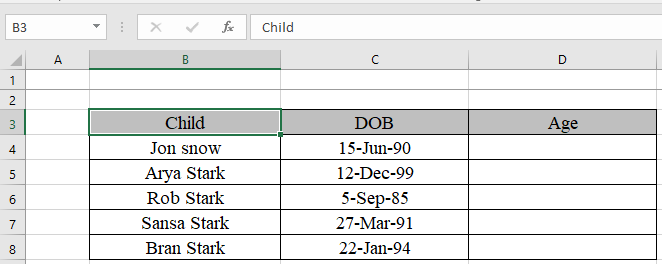We will use a combination of INT and TODAY function to find the age of chidren.
Use the formula in D4 cell to get Jon snow’s age.

Formula to find the age in Excel:

=INT((TODAY()-C4)/365)

Explanation:
TODAY()-C4 calculates the no. of days between today and date of birth.

We divide the number 365 to get the years between today and date of birth. INT function takes out the integer part from the value.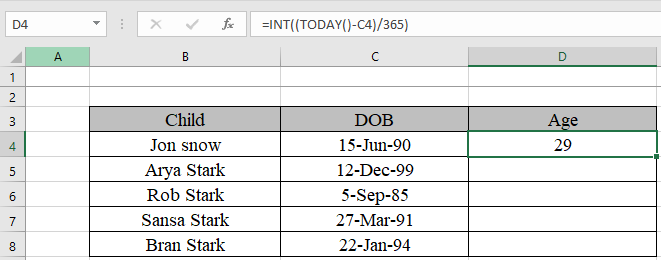Yes. We got Jon Snow’s age..

Copy the formula in other cells, select the cells taking the first cell where the formula is already applied, use shortcut key Ctrl+D to find the age of rest of the children.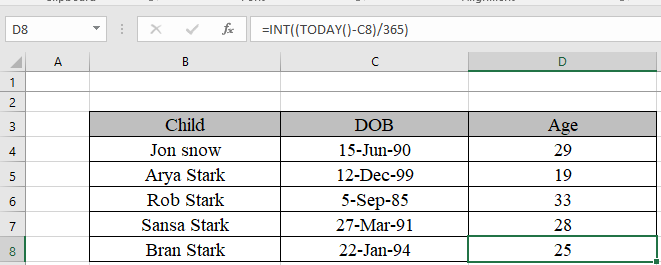As you can see we got the age of Ned Stark’s children just by simple formulation. Whenever the sheet is used the age will be updated.
There’s one more way using DATEIF function.

DATEDIF function returns the number of years, months and days between the given two dates.
Syntax:

=DATEDIF(start_date, end_date, unit)

We will use the formula to get the age of children

Formula:

=DATEDIF(C4,TODAY(),"y")

Date of birth is the start date in the C4 cell.
Today() function is used to get Today’s date as an end date.
“Y” to get the age in years.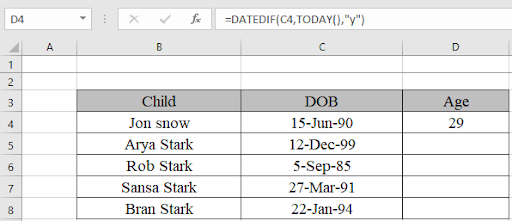Yes. We got Jon Snow’s age using the DATEDIF function.

Copy the formula in other cells, select the cells taking the first cell where the formula is already applied, use shortcut key Ctrl+D to find the age of rest of the children.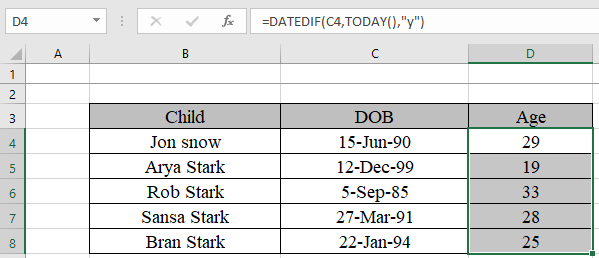Hope you understood how to calculate the age from date of birth. You can perform these tasks in Excel 2013 and 2010 workbook. Please share your any query below in the comment box. We will assist you.

Popular Articles:

50 Excel Shortcuts to Increase Your Productivity

How to use the VLOOKUP Function in Excel

How to use the COUNTIF function in Excel

How to use the SUMIF Function in Excel

1.Thanks for thehelp

2.The result comes as value

3.How to convert in Days if my Date of birth is 1985/06/21

4.you can use the following function for calculating the correct age in years, months and days.
=DATEDIF(A1,A2,"y") & "years, " & DATEDIF(A1,A2, "ym") & " months, " & DATEDIF(A1,A2, "md") & " days "

5.Hi. I want excel to work out what someone's age will be at an event on 9 August 2014, in order to assist allocating kids to their correct age group activities. Thanks

6."Hi Rebouche,

I am not familiar with mail merge, but perhaps someone else will be able to help you with why it changesthe value back to fifteen decimals.

The value returned by the formula will either be """" (blank) or will be a number to one decimal place.

However, as a workaround, you could perhaps copy and paste the answers to values, thus removing the formulae, and leaving only the values (to one decimal place), before you mail merge.

Would that work for you?

Alan.
"

7."Hi Alan,
Using your corrected formula, and when I pass to my document, I am always getting 15 (fifteen) decimals instead of (1) one.
Meawhile, I replaced the (,) by (;).
Thanking you,
Rebouche"

8."Hi Rebouche,

You are right - it doesn't!

I copied your formula from your post, and just put the ROUND outside of it. I should have done it in Excel and pasted to here:

=IF(A1=0,"""",ROUND(((B1-A1)+1)/365,1))

Does that work?

Alan."

9."Hi Alan,
Unfortunately, it does not work with me.
Rebouche"

10."Hi Rebouche,

If you change your formula to:

=Round(IF(A1=0;"""";((B1-A1)+1/365),1)

then this will change the actual value to be rounded to one decimal place.

Does that work for you?

Alan."

11."Hi Alan,
It is mail merge between Word and Excel, and my target is to select people using age with one decimal only (as mentioned already).
For example, When I am inserting the date of birth in A1(which is = 14 June 1962) and in B1 (=NOW()) and in another cell, I am using the follwing formula: =IF(A1=0;"""";((B1-A1)+1/365). I am getting the following result 41.25018031 formatted in number with one decimal only 41.3.
The problem is when I am reporting this age to my document (Word) the result is as follow: 41.2501533878744171 but I need only to have 41.3 nothing else ... Can you help to resolve this problem by keeping at least the same formula mentioned above.
Thanks "

12."Hi Rebouche,

Please can you clarify a little.

The problem is that a month is not a well defined period of time (can be anything from 28 days to 31 days inclusive).

Therefore, it is difficult to use a 'month' as a measure of time (as opposed to an absolute time reference for which it is very well defined).

The same problem arises for a 'year' - as discussed above. A year can be either 365 or 366 days, and is therefore, not well defined either as a measure of time. What exactly would you mean by 0.3 of a year?

Excel has functions that will 'standardise' those period, and resolve the issue that you are encountering by assuming that every year has 360 days, and every month exactly 30 days. See Excel help on the DAYS360 function.

In general, I would suggest that you steer clear of representing someones age as ""40.3"" if at all possible. Giving the age to three significant figures, implicitly leads the reader to assume a level of accuracy that may not be borne out of you ask a selection of people what it means.

If you have to do this, then you need to define your units explicitly for the reader of the report (and other users of the spreadsheet model) before you start so that everyone knows exactly what you mean.

Does that help? Post back if you want more help, but I suggest that the 360 day year has to be the way to go for you, even though it leads to errors close to a person's birthday.

Alan."

13."Dear Sirs,
By using the formula =INT((TODAY()-A1)/365.25), I am getting a whole number as age (e.g. 40).
Now if I want to have the number of month also (Years+Months e.g. 40.3) and when I want to merge with word, the same age will appear with one decimal only (e.g. 40.3) and not fifteen (15) decimals (as 40.29847562534987)
Which formula I have to use.
Many Thanks."

14."From Excel help:

When you enter a date in a cell and you enter only two digits for the year, Excel interprets the year as follows:

The years 2000 through 2029 if you type 00 through 29 for the year. For example, if you type 5/28/19, Excel assumes the date is May 28, 2019.

The years 1930 through 1999 if you type 30 through 99 for the year. For example, if you type 5/28/98, Excel assumes the date is May 28, 1998.
If you are using Microsoft Windows 98 or Microsoft Windows 2000, you can, without the assistance of your system administrator, change the way two-digit years are interpreted."

15."Hi Chris,

That is nothing to do with Excel directly.

You have your computer set up to make that assumption, and Excel has followed your instructions accordingly.

Check out your date settings under Regional Settings.

Alan."

16."You can calculate a persons Age in years using:

=DATEDIF(A1,NOW(),""y"") "

17.When inputting a birthdate that is before 1/1/1930 (i.e. 12/31/29), excel defaults the year as 2029. Why is this and is there a fix or a setting?

Terms and Conditions of use

The applications/code on this site are distributed as is and without warranties or liability. In no event shall the owner of the copyrights, or the authors of the applications/code be liable for any loss of profit, any problems or any damage resulting from the use or evaluation of the applications/code.